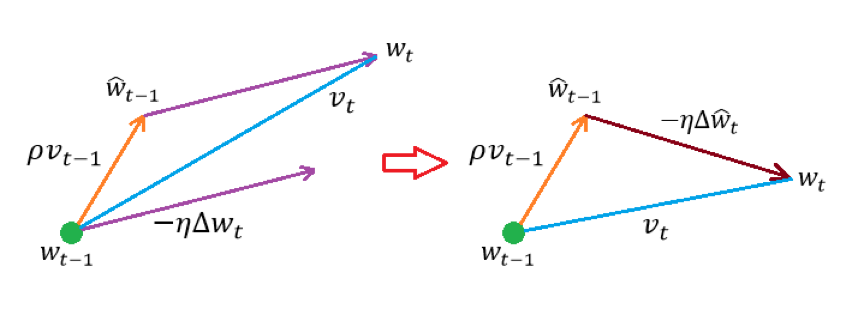• 更新方向不是简单地取为梯度
• 学习速率不是简单地取为常值

• 权值矩阵：$w^{\left( i - 1 \right)}$的维度为$n_{i - 1} \times n_{i}$
• 输出向量：$v^{\left( i - 1 \right)}$的维度为$N \times n_{i - 1}$
• 局部梯度：$\delta^{\left( i \right)}$的维度为$N \times n_{i}$

• 接收欲更新的参数并进行相应处理的方法
• 利用梯度和自身属性来更新参数的方法
• 在完成参数更新后更新自身属性的方法

# Vanilla Update

Vanilla 在机器学习中常用来表示“朴实的”、“平凡的”，换句话说、Vanilla
Update 和最普通的梯度下降法别无二致，亦即：

# Momentum Update

Vanilla Update 的缺点是比较明显的：以 MBGD 为例，它每一步迭代中参数的更新是完全独立的、亦即第t步参数的更新方向只依赖于当前所用的 batch，这在物理意义上是不太符合直观的。可以进行如下设想：

• 将损失函数的图像想象成一个山谷、我们的目的是达到谷底
• 将损失函数某一点的梯度想象成该点对应的坡度
• 将学习速率想象成沿坡度行走的速度

• 认为训练刚开始时的梯度会比较大而训练后期时梯度会变小，通过逐步调大$\rho$、我们能够使更新的步伐一直保持在比较大的水平
• 认为当我们接近谷底时、我们应该尽量减少“动力”带来的影响而保持原有的方向前进。这是因为如果每一步都直接往谷底方向走（亦即运动仅受动力影响）的话、就会很容易由于动力大小难以拿捏而引发震荡

# Nesterov Momentum Update

Nesterov Momentum Update 的核心思想在于想让算法具有“前瞻性”。简单来说、它会利用“下一步”的梯度而不是“这一步”的梯度来合成出最终的更新步伐（所谓更新步伐、可以直观地理解为“更新方向$\times$更新幅度”）。可以通过下图来直观地认知这个过程：• 起点$w_{t - 1}$处的行进速度$\rho v_{t - 1}$
• 中继点${\hat{w}}_{t - 1}$处的更新步伐$- \eta\Delta w_{t}$$w_{t - 1}$处的负梯度与学习速率的乘积）

• 起点$w_{t - 1}$处的行进速度$\rho v_{t - 1}$
• 中继点${\hat{w}}_{t - 1}$处的更新步伐$- \eta\Delta{\hat{w}}_{t}$${\hat{w}}_{t - 1}$处的负梯度与学习速率的乘积）

# RMSProp

RMSProp 方法与 Momentum 系的方法最根本的不同在于：Momentum 系算法是通过搜索更优的更新方向来进行优化、而 RMSProp 则是通过实时调整学习速率来进行优化。具体而言、它的优化公式为：

• 中间变量$\nabla^{2}$，它是从算法开始到当前步骤的所有梯度的某种“累积”
• 衰减系数$\rho$，它反映了比较早的梯度对当前梯度的影响、$\rho$越小则影响越小